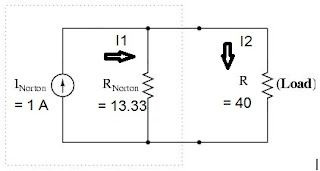## Sunday, March 17, 2013

### Simple Example of Network Analysis

Last time, I have already imparted to you about the theoretical aspects of Network Analysis here in my simple Electrical Engineering educational site. Today, I will just give you some simple example for you to appreciate the last topic I had posted over a year ago. :)

Take a look at the simple electric circuits below. If you have a voltage divider with an external resistance, you could do this by using Ohm' Law and calculating the parallel resistance of R2 and R(Load) and then the voltage divider itself. The simpler method that you can use is the Thevenin's theorem which enables you to calculate quickly the effect of any load.Simple Circuit using Thevenin's Theorem

Considering you have R(Load) equivalent to 40 ohms is in open circuit condition. We can now calculate the equivalent Thevenin resistance.

Therefore,
R (Thevenin) = R1R2 / R1+R2 = 20X40/ 20+40 = 13.33 ohms.

Also, you can calculate the voltage across R2 at no load using the voltage division method:
E(Thevenin) = Ein R2 / R1+R2 = 20x40 /20+40 = 13.33 Volts

When the resistance load or R(Load) of 40 ohms  is added as shown above, by using Ohms Law for simple series circuits you can now obtain the ouput voltage:

E(Load) = E(Thevenin) x R (Load) / R(Thevenin) + R(Load) = 13.33 x 40/ 13.33+40 = 10 volts - Ans.

Using other method like Norton's Theorem is also a good method to used in this given problem. Now, instead of open circuit condition, let's make it short circuit condition at the load side R(Load).Simple Circuit Using Norton's Theorem
In this condition, we can calculate the current when R2 is shorted. Let's called it I (Norton):
I (Norton) = Ein / R1 = 20V / 20 Ohms = 1 Ampere

Therefore, the equivalent circuit will comes out like this:Norton's Equivalent Circuit
From Thevenin's equivalent circuit above for R1 and R2, the parallel combination was computed as 13.33 ohms. We know that the voltage across R(Norton) and R(Load) are the same when R(Load) is connected but the total current is still 1 Ampere.

Let's make an analysis now:

We can get load voltage E (out) in two ways:
1---> E(out) = I1 x R(Norton) = I1 x 13.33
2---> E(out) = I2 x R(Load) = I2 x 40

Then, we can equate the two equations above:
3--->I1 x 13.33=I2 x 40

Using Kirchoff's current law,
I(total) = I1 + I2 = 1 Ampere

We can now solve I1 by substitution method: (Remember, you should used you math technique sometimes to solve particular problems is Electric Circuits)

We can substitute I2 value: I2 = I(total)-I1 or 1- I1 to equation 3.
I1 x 13.33 = (1-I1) 40
I1 = 0.75 Ampere

Since, I(total) is 1 Ampere, therefore I2 = 1-0.75 = 0.25 Ampere
Finally, we can calculate E(out) in either equation 1 or 2 above:

E(out) = I1 x R(Norton) or I2 x R(Load) = 0.25 x 40 = 10 volts - Ans.

The two methods used either Thevenin's or Norton's still have same results obtained. This is the magic of Network Analysis!